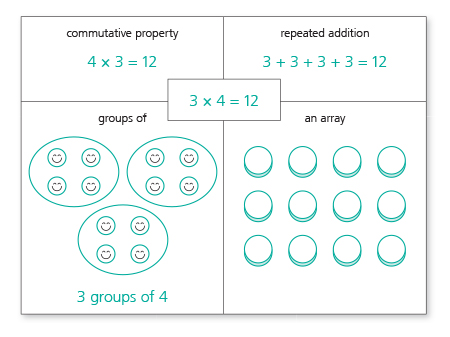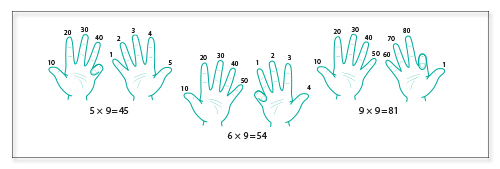# Six helpful strategies for learning times tables

20 April 2017

#### Recent postsEveryone remembers learning their times tables. Whether you got them straight away or still find yourself reaching for the calculator, here are six practical suggestions for ways that you can help children grasp multiplication and division facts in no time at all.

Instant recall of times tables is essential to success in all areas of maths. In fact, you could say that times tables are to maths what phonics is to English. Without them, children lack the basic tools to decode even simple calculations, never mind complex real-world problems. Put simply, if children are fluent in the basic multiplication and division facts, then their working memory is freed up from having to make simple calculations, and can think more deeply about the conceptual underpinnings of a problem.

As with most things that are worth learning, repetition is the key. It is essential therefore to make times tables practice part of your everyday teaching, even after the basic order has been learnt. Though chanting the times tables individually or as a whole class is a good place to start, there are many additional strategies that will make learning the times tables easier for pupils. Below are six different approaches that you can use to help children master their times tables with ease.

1. Looking for patterns

There is some debate surrounding the best order in which to teach the times tables. However, it is generally agreed that it is preferable to start with 2, 5 and 10, as the patterns within them are easy for young children to grasp. In the 2 and 10 times tables the numbers are all even. In fact, any number multiplied by an even number will be even. In the 10 times table all the numbers end in 0 and in the 5 times table all the numbers end in 5 or 0.

Once they have mastered the 2, 5 and 10 times tables, children should find it easier to move on to 4 and 8. Again, point out the patterns. Show pupils that the answers for the 4 times table are the answers to the 2 times table doubled: for example, 3 × 4 = 3 × 2 × 2. Likewise, the answers for the 8 times table are the answers to the 4 times table doubled: for example, 5 × 8 = 5 × 4 × 2.

When they come to the 3, 6 and 9 times tables, children will see that the digits of each answer add together to make 3, 6 or 9, or a multiple of those numbers. In the 9 times table they will also see a pattern in the tens and ones, with the tens increasing and the ones decreasing as you go up the times table.

2. The rule of commutativity

The rule of commutativity is useful for children learning times tables because it shows that they know more than they might think. Thanks to commutativity, you can tell pupils that when they learn a multiplication fact, they also learn the following three additional facts:

• When writing a multiplication, the numbers being multiplied can be written in any order. So if a child knows that 2 × 8 = 16, they also know that 8 × 2 = 16.
• For every multiplication fact there are related division facts. So if a child knows that 2 × 8 = 16, they also know that 16 ÷ 2 = 8.
• Lastly, if a child knows that 16 ÷ 2 = 8, they also know that 16 ÷ 8 = 2.

3. Oral activities

Songs, rhymes, raps and stories are all useful tools for helping children memorise multiplication facts. You can find lots of musical inspiration on websites like YouTube, such as a hip-hop version of the 5 times table, a reggae rendition of the 2 times table or a times tables quiz based on ‘The Twelve Days of Christmas’. Alternatively, you could encourage pupils to make up their own songs and rhymes, perhaps even with added dance moves.

As far as stories go, the classic shopping memory game can easily be adapted to enable children to practise their times tables. Start by getting pupils to sit in a circle, before saying the following phrase aloud: ‘I went to the shop and I bought …’, followed by the first number in the times table sequence you want to practise, ‘two’, for example, together with some items, say ‘apples’. Then get the first child in the circle to say the same phrase, ‘I went to the shop and I bought two apples’, followed by ‘and’, the next number in the times table sequence and another group of items. The children then take turns going round the circle, repeating the items on the shopping list and adding more items according to the times table you have chosen.

4. Practical activities

It’s important that children regularly write out the times tables, in addition to saying them out loud. You should also try to incorporate physical movement when reciting the times tables with pupils. For example, when practising the 5 times table, ask the pupils to give their answers by flashing 5 fingers the appropriate number of times. When a child struggles with a particular multiplication fact, encourage them to make a flashcard with the question on one side and the answer on the other. They can then take it home and stick it on their fridge or bedroom wall as a helpful reminder.

You could also ask the children to make posters, collages, displays and other artwork that illustrates a particular times tables or group of times tables. For example, the illustration below is a useful way of showing the variety of methods for thinking about individual multiplication facts. Children could make their own versions of this as a poster, using stickers, buttons, beads and even types of food for groups and arrays. Other creative options include getting children to make times table fortune tellers, multiplication flower wheels and customised times tables booklets.5. Games

Given that the ultimate goal when learning times tables is instant recall, beat-the-clock games can be a great way of improving children’s speed and fluency. Start by timing pupils to write out times tables or complete short tests, such as those in Schofield & Sims Times Tables Tests, encouraging them to improve on their time in each instance. When children have learned all the multiplication tables, they could even race each other to see who can fill out a multiplication square the fastest.

Another great game that will help children to become confident with the times tables, even when under pressure is Fizz Buzz. Sit the pupils in a circle and ask them to take turns counting aloud, starting with 1. The catch is that instead of saying some numbers, the pupils will have to say either ‘fizz’ or ‘buzz’. Any number that is a multiple of 3 should be replaced with ‘fizz’ and any number that is a multiple of 5 should be replaced with ‘buzz’. When a pupil comes to a number that is a multiple of both 3 and 5, such as 15, they must say ‘fizz buzz’. You can increase the difficulty by introducing a third option using the word ‘woof’ or by asking children to leave the circle if they make a mistake – thereby increasing the frequency with which the remaining participants have to answer.

6. Tricks

Even after you have tried all the activities listed above, it is possible that some children will continue to struggle with a particular times table, in which case, there are a few final tricks that can help. Perhaps the most well-known example is the trick for finding the answers in the 9 times table, up to 9 × 9, whereby you use your fingers to demonstrate the changes in the tens and ones, as the illustration below demonstrates.There are more tricks to learn for the higher times tables. For example, in the 11 times table, multiples of  11 that are less than one hundred are simply the multiplied digit repeated: so 2 × 11 = 22,  3 × 11 = 33, 4 × 11 = 44, and so on. Multiples of 11 that are greater than 100 also follow a pattern that, like the above pattern for the 9 times table, relies on place value. Take 12 × 11, for example. First, take the number you wish to multiply by 11: 12. Place its first digit in the hundreds column and its last digit in the ones column: 1_2. Then add those digits together: 1 + 2 = 3, and place that number in the tens column.

For many children 7 x 8 poses a particular problem, combining as it does both the 7 and 8 times tables. Fortunately, there is a very simple way of remembering this times table. Simply place the answer first and count on: 56 = 7 x 8. Easy! Another trick that can be helpful with the harder times tables is using square numbers, as the numbers can be used as a framework for mastering other multiplication facts. For example, if a child needs to answer 7 × 6 and knows that 6 × 6 = 36, they can work out the answer by adding 6 to 36.